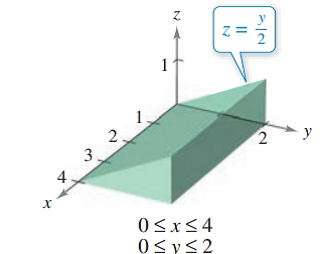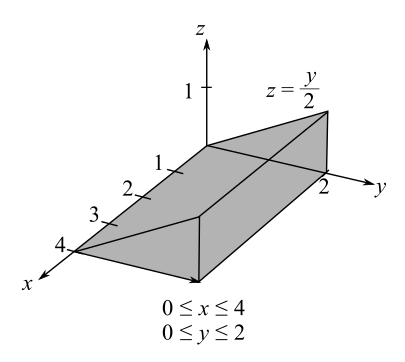Chapter 7.9, Problem 13E### Calculus: An Applied Approach (Min...

10th Edition
Ron Larson
ISBN: 9781305860919

#### Solutions

Chapter
Section### Calculus: An Applied Approach (Min...

10th Edition
Ron Larson
ISBN: 9781305860919
Textbook Problem
1 views

# Finding the Volume of a Solid Region In Exercises 13-20, use a double integral to find the volume of the solid region.To determine

To calculate: The volume of solid region that shown below,Explanation

Given information:

The provided solid region is

Formula used:

The procedure to calculate volume of surface z=f(x,y),

Step-1: Write the equation of surface in the form z=f(x,y)

Step-2: Sketch the projected region R in the x-y-plane.

Step-3: Determine the order of limits of integration.

Step-4: Evaluate the volume of solid region,

Volume=mnabf(x,y)dxdy

Here, the projected region is R:

myn and axb

Calculation:

Consider graph of solid region,

The function of upper surface written as follow,

f(x,y)=y2

The projected region R 0x4,0y2 in the x-y-plane plotted as follow,

The bounds for x are 0x4 and bounds for y are 0y2.

The volume for the solid region is,

Volume=0402(y2)dydx

For order of limit the bounds for y are 0y12 and bounds for x are 0xy3

### Still sussing out bartleby?

Check out a sample textbook solution.

See a sample solution

#### The Solution to Your Study Problems

Bartleby provides explanations to thousands of textbook problems written by our experts, many with advanced degrees!

Get Started

#### Evaluate the integral. 12. 2x3x3+3xdx

Single Variable Calculus: Early Transcendentals

#### For , an upper bound estimate for s − s6 (where s6 is the sixth the series does not converge

Study Guide for Stewart's Single Variable Calculus: Early Transcendentals, 8th